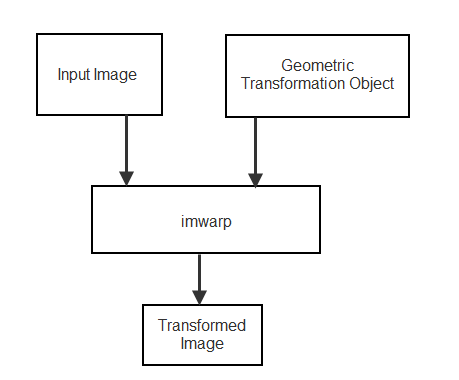Documentation

This is machine translation

Mouseover text to see original. Click the button below to return to the English version of the page.

2-D and 3-D Geometric Transformation Process Overview

To perform a 2-D or 3-D geometric transformation, first create a geometric transformation object that stores information about the transformation. Then, pass the image to be transformed and the geometric transformation object to the `imwarp` function. You optionally can provide spatial referencing information about the input image to `imwarp`.Create Geometric Transformation Object

Different types of geometric transformation objects store different information about the transformation.

There are several ways to create a geometric transformation object.

Approach to Create Tranformation Object`affine2d``affine3d``projective2d````geometricTransform2d / geometricTransform3d```Other Transformation Objects
Define Transformation MatrixXXX
Define Custom Point-Wise Mapping Function   X
Estimate Transformation from Control Point PairsX X X
Estimate Transformation Using Similarity OptimizationXX
Estimate Transformation Using Phase CorrelationX

Define Transformation Matrix

If you know the transformation matrix for the geometric transformation you want to perform, then you can create a `affine2d`, `projective2d`, or `affine3d` geometric transformation object directly. For more information about creating a transformation matrix, see Matrix Representation of Geometric Transformations.

The following example defines the transformation matrix for a 2-D translation and creates an `affine2d` geometric transformation object.

```xform = [ 1 0 0 0 1 0 40 40 1 ]; tform_translate = affine2d(xform); ```
```tform_translate = affine2d with properties: T: [3x3 double] Dimensionality: 2```

Define Custom Point-Wise Mapping Function

If you have an inverse point-wise mapping function, then you can define a custom 2-D and 3-D geometric transformation using the `geometricTransform2d` and the `geometricTransform3d` objects respectively.

The following example specifies an inverse mapping function that accepts and returns 2-D points in packed (x,y) format. Then, the example creates a `geometricTransform2d` custom geometric transformation object.

`inversefn = @(c) [c(:,1)+c(:,2),c(:,1).^2]`
```inversefn = function_handle with value: @(c)[c(:,1)+c(:,2),c(:,1).^2]```
`tform = geometricTransform2d(inversefn)`
```tform = geometricTransform2d with properties: InverseFcn: @(c)[c(:,1)+c(:,2),c(:,1)-c(:,2)] ForwardFcn: [] Dimensionality: 2```

Similarly, the following example creates a `geometricTransform3d` custom geometric transformation object using the inverse mapping function. The example specifies an inverse mapping function that accepts and returns 3-D points in packed (x,y,z) format.

`inversefn = @(c)[c(:,1)+c(:,2),c(:,1)-c(:,2),c(:,3).^2]`
```inversefn = function_handle with value: @(c)[c(:,1)+c(:,2),c(:,1)-c(:,2),c(:,3).^2]```
`tform = geometricTransform3d(inversefn)`
```tform = geometricTransform3d with properties: InverseFcn: @(c)[c(:,1)+c(:,2),c(:,1)-c(:,2),c(:,3).^2] ForwardFcn: [] Dimensionality: 3```

Estimate Transformation from Control Point Pairs

You can create a geometric transformation object by passing two sets of control point pairs to the `fitgeotrans` function. The `fitgeotrans` function automatically estimates the transformation from these points and returns one of the geometric transformation objects.

Different transformations require a varying number of points. For example, affine transformations require three non-collinear points in each image (a triangle) and projective transformations require four points (a quadrilateral).

This example passes two sets of control points to `fitgeotrans`, which returns an `affine2d` geometric transformation object.

```movingPoints = [11 11;21 11; 21 21]; fixedPoints = [51 51;61 51;61 61]; tform_cpp = fitgeotrans(movingPoints,fixedPoints,'affine') ```
```tform_cpp = affine2d with properties: T: [3x3 double] Dimensionality: 2```

Estimate Transformation Using Similarity Optimization

If you have a fixed image and a moving image that are slightly misaligned, then you can use the `imregtform` function to estimate an affine geometric transformation that aligns the images. `imregtform` optimizes the mean squares or Mattes mutual information similarity metric of the two images, using a regular step gradient descent or one-plus-one evolutionary optimizer. For more information, see Create an Optimizer and Metric for Intensity-Based Image Registration.

Estimate Transformation Using Phase Correlation

If you have a fixed image and a moving image that are severely misaligned, then you can use the `imregcorr` function to estimate an affine geometric transformation that improves the image alignment. You can refine the resulting transformation by using similarity optimization.

Perform the Geometric Transformation

After you define the transformation in a geometric transformation object, perform the transformation by using the `imwarp` function. When calling the function, specify the image to be transformed and the geometric transformation object.

`imwarp` uses the geometric transformation to map coordinates in the output image to the corresponding coordinates in the input image (inverse mapping). Then, `imwarp` uses the coordinate mapping to interpolate pixel values within the input image and compute the output pixel value.